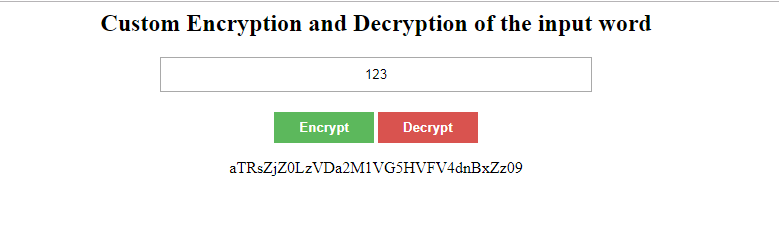Google trends Public Holidays Coupon Code Code Compiler

# Encrypt and Decrypt word using openssl_encrypt and openssl_decrypt

Jan 30, 2019Encrypt and Decrypt password. The php manual is currently lacking documentation for the “openssl_encrypt” and “openssl_decrypt” functions, so it took me awhile to piece together what I needed to do to get these functions working as a replacement for mcrypt, which has been unmaintained since 2003. Hopefully this will help you get to where you need to go with encrypting and decrypting your word.

First You need to create 2 keys ( secret_key and secret_iv )

PHP Code:

``````
// Encryption Method
function encrypt(\$string) {
\$output = false;
\$encrypt_method = "AES-256-CBC";

\$secret_key = 'regYUDkbbdsc78yyBJB7J';
\$secret_iv = 'UIBbsvvjJHWUkubBubIVU';

// hash
\$key = hash('sha256', \$secret_key);

\$iv = substr(hash('sha256', \$secret_iv), 0, 16);

\$output = openssl_encrypt(\$string, \$encrypt_method, \$key, 0, \$iv);
\$output = base64_encode(\$output);

return \$output;
}

// Decryption Method
function decrypt(\$string) {
\$output = false;

\$encrypt_method = "AES-256-CBC";
\$secret_key = 'regYUDkbbdsc78yyBJB7J';
\$secret_iv = 'UIBbsvvjJHWUkubBubIVU';

// hash
\$key = hash('sha256', \$secret_key);

// iv - encrypt method AES-256-CBC expects 16 bytes - else you will get a warning
\$iv = substr(hash('sha256', \$secret_iv), 0, 16);

\$output = openssl_decrypt(base64_decode(\$string), \$encrypt_method, \$key, 0, \$iv);

return \$output;
}
\$msg = '';
if(isset(\$_POST['btn_encrypt'])){
\$str = \$_POST['str'];
\$result = encrypt(\$str);
\$msg = \$result;
}
else if(isset(\$_POST['btn_decrypt'])){
\$str = \$_POST['str'];
\$result = decrypt(\$str);
\$msg = \$result;
}
```
```

CSS Code:

``````
.wraper{
width:80%;
margin:auto;
text-align:center
}
.input-field{
width: 40%;
height: 35px;
display: block;
text-align:center;
margin: auto;
}
.btn-group{
margin-top: 20px;
}
.btn-group input{
border: none;
color: #fff;
font-weight: bold;
}
.success{
background:#5CB85C
}
.danger{
background:#D9534F
}
```
```

HTML Code:

``````
<div class="wraper">
<div class="form-group">
<h2>Custom Encryption and Decryption of the input word</h2>
<form action="" method="post">
<input type="text" name="str" value="<?php echo @\$_POST['str']; ?>" class="input-field" placeholder="Enter your encrypted string to decrypt / word to encrypt..">
<div class="btn-group">
<input type="submit" class="success" name="btn_encrypt" value="Encrypt">
<input type="submit" class="danger" name="btn_decrypt" value="Decrypt">
</div>
</form>
<?php echo \$msg; ?>
</div>
</div>
```
```

## Recent Article

Copyright 2023. All rights are reserved